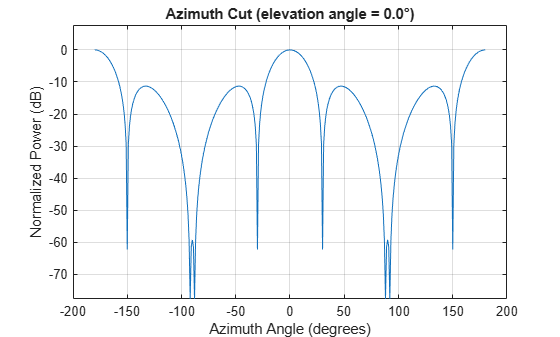phased.ArrayResponse

Sensor array response

Description

The ArrayResponse object calculates the complex-valued response of a sensor array.

To compute the response of the array for specified directions:

1. Define and set up your array response calculator. See Construction.

2. Call step to estimate the response according to the properties of phased.ArrayResponse. The behavior of step is specific to each object in the toolbox.

Note

Starting in R2016b, instead of using the step method to perform the operation defined by the System object™, you can call the object with arguments, as if it were a function. For example, y = step(obj,x) and y = obj(x) perform equivalent operations.

Construction

H = phased.ArrayResponse creates an array response System object, H. This object calculates the response of a sensor array for the specified directions. By default, a 2-element uniform linear array (ULA) is used.

H = phased.ArrayResponse(Name,Value) creates object, H, with each specified property Name set to the specified Value. You can specify additional name-value pair arguments in any order as (Name1,Value1,...,NameN,ValueN).

Properties

 SensorArray Handle to sensor array used to calculate response Specify the sensor array as a handle. The sensor array must be an array object in the phased package. The array can contain subarrays. Default: phased.ULA with default property values PropagationSpeed Signal propagation speed Specify the propagation speed of the signal, in meters per second, as a positive scalar. Default: Speed of light WeightsInputPort Add input to specify weights To specify weights, set this property to true and use the corresponding input argument when you invoke step. If you do not want to specify weights, set this property to false. Default: false EnablePolarization Enable polarization simulation Set this property to true to let the array response simulate polarization. Set this property to false to ignore polarization. This property applies only when the array specified in the SensorArray property is capable of simulating polarization. Default: false

Methods

 step Calculate array response of sensor array
Common to All System Objects
release

Allow System object property value changes

Examples

collapse all

Calculate array response for a 4-element uniform linear array (ULA) in the direction of 30 degrees azimuth and 20 degrees elevation. Assume the array's operating frequency is 300 MHz.

Construct ULA and ArrayResponse System objects

fc = 300e6;
c = physconst('LightSpeed');
array = phased.ULA(4);
response = phased.ArrayResponse('SensorArray',array);
resp = response(fc,[30;20])
resp = 0.2768

Plot the array response in dB

Plot the normalized power in db as an azimuth cut at 0 degrees elevation.

pattern(array,fc,[-180:180],0,'PropagationSpeed',c,'CoordinateSystem','rectangular','Type','powerdb')References

 Van Trees, H. Optimum Array Processing. New York: Wiley-Interscience, 2002.